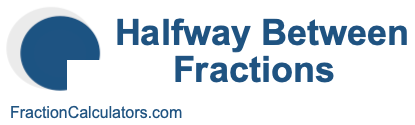Halfway Between Fractions CalculatorWelcome to the Halfway Between Fractions Calculator. This calculator can calculate the exact halfway point between two fractions.

In other words, use this calculator if you have two fractions and want to find the fraction that is exactly halfway between them.

To get started, please enter your set of fractions below.

Halfway between
/
and
/

Here are some examples of what the Halfway Between Fractions Calculator can solve for you.

What fraction is halfway between 1/4 and 1/2?

What fraction is halfway between 1/10 and 3/5?

What fraction is halfway between 1/2 and 3/4?

What fraction is halfway between 1/8 and 1/4?

What fraction is halfway between 1/3 and 1/2?

What fraction is halfway between 1/4 and 3/8?

What fraction is halfway between 1/3 and 2/3?

What fraction is halfway between 2/3 and 3/4?

What fraction is halfway between 2/5 and 8/15?

What fraction is halfway between 3/4 and 7/8?